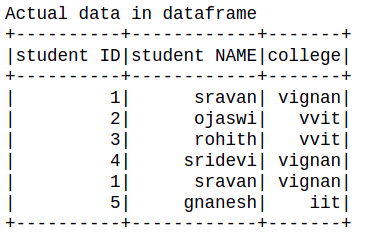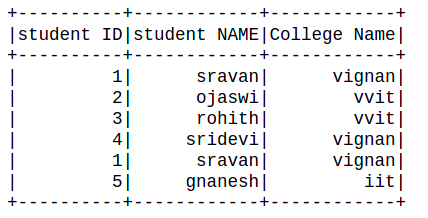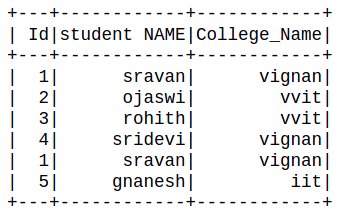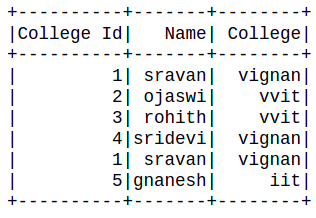# How to rename multiple columns in PySpark dataframe ?

• Last Updated : 04 Jul, 2021

In this article, we are going to see how to rename multiple columns in PySpark Dataframe.

Before starting let’s create a dataframe using pyspark:

## Python3

 `# importing module``import` `pyspark``from` `pyspark.sql.functions ``import` `col`` ` `# importing sparksession from pyspark.sql module``from` `pyspark.sql ``import` `SparkSession`` ` `# creating sparksession and giving an app name``spark ``=` `SparkSession.builder.appName(``'sparkdf'``).getOrCreate()`` ` `# list  of students  data``data ``=` `[[``"1"``, ``"sravan"``, ``"vignan"``],``        ``[``"2"``, ``"ojaswi"``, ``"vvit"``],``        ``[``"3"``, ``"rohith"``, ``"vvit"``],``        ``[``"4"``, ``"sridevi"``, ``"vignan"``],``        ``[``"1"``, ``"sravan"``, ``"vignan"``],``        ``[``"5"``, ``"gnanesh"``, ``"iit"``]]`` ` `# specify column names``columns ``=` `[``'student ID'``, ``'student NAME'``, ``'college'``]`` ` `# creating a dataframe from the lists of data``dataframe ``=` `spark.createDataFrame(data, columns)`` ` `print``(``"Actual data in dataframe"``)`` ` `# show dataframe``dataframe.show()`

Output:Method 1: Using withColumnRenamed.

Here we will use withColumnRenamed() to rename the existing columns name.

Syntax: withColumnRenamed( Existing_col, New_col)

Parameters:

• Existing_col: Old column name.
• New_col: New column name.

Example 1: Renaming single columns.

## Python3

 `dataframe.withColumnRenamed(``"college"``, ``                            ``"College Name"``).show()`

Output:Example 2: Renaming multiple columns.

## Python3

 `df2 ``=` `dataframe.withColumnRenamed(``"student ID"``,``                                  ``"Id"``).withColumnRenamed(``"college"``,``                                                          ``"College_Name"``)``df2.show()`

Output:Method 2: Using toDF()

This function returns a new DataFrame that with new specified column names.

Syntax: toDF(*col)

Where, col is a new column name

In this example, we will create an order list of new column names and pass it into toDF function.

## Python3

 `Data_list ``=` `[``"College Id"``,``" Name"``,``" College"``]``new_df ``=` `dataframe.toDF(``*``Data_list)``new_df.show()`

Output:My Personal Notes arrow_drop_up